Numbers, Theory of

Numbers, Theory of

the science of the integers, or whole numbers. The concept of integer and the concept of arithmetical operations on numbers have been known since antiquity and are one of the first mathematical abstractions.

The natural numbers, that is, the positive integers, 1, 2, 3, . . . , the properties of natural numbers, and the operations on such numbers occupy a special place among the integers, that is, the numbers . . . , –3, –2, –1, 0, 1, 2, 3, . . . . All natural numbers greater than unity fall into one of two classes; the first class includes numbers that have exactly two natural divisors, namely unity and themselves, while the second class includes all other numbers. The numbers of the first class have come to be called prime numbers, and the numbers of the second class, composite numbers. The properties of prime numbers and their relationship to all natural numbers were studied by Euclid (third century B.C.). If the prime numbers are written in sequence, it can be seen that their relative density decreases: there are four prime numbers, or 40 percent, in the first ten numbers; 25, or 25 percent, in the first hundred; 168, or about 17 percent, in the first thousand; 78,498, or about 8 percent, in the first million, and so forth; however, there are infinitely many prime numbers (Euclid).

The prime numbers include some pairs the difference between whose members is equal to two (twin primes), but whether such pairs are infinite or finite in number has not been proved.

Euclid believed it obvious that all the natural numbers could be obtained by multiplying only prime numbers and that every natural number could be represented uniquely (to within the order of the factors) as the product of prime numbers. Thus, the prime numbers form the multiplicative foundation of the natural numbers. The first problems of prime numbers were concerned with how frequently primes occur in the natural sequence and how far they are from each other. The study of the distribution of prime numbers led to the creation of an algorithm (rule) that makes it possible to compile tables of primes. The sieve of Eratosthenes (third century B.C.)is such an algorithm. In Elements, Euclid indicated a method of finding the greatest common divisor of the two numbers (Euclid’s algorithm), a consequence of which is the theorem of the unique factorization of the natural numbers into prime factors.

The problem of integral solutions of different types of equations also dates to antiquity. The simplest equation in integral values of the unknowns is the linear equation

aX + bY = c

where a, b, and c are pairwise relatively prime integers. The solution of the equation aX+ b Y = 1 is found by using Euclid’s algorithm, from the solution of which all solutions of the original equation are then obtained. Another equation in integral values of the unknowns is X2 + Y2 = Z2 (the solutions X = 3, Y = 4, Z = 5 are Pythagorean numbers), all of whose solutions in integers were given in Elements (Book X, Proposition 29):

X = r2q2Y= 2rq Z = r2 + q2

where r and q are integers. Euclid was also familiar with the equation aX2 + 1 = Y2, which subsequently became known as Pell’s equation (seePELLS EQUATION). In Elements (Book X, Proposition 9), Euclid provided all the solutions of this equation, beginning with the smallest solution, for the case a = 2. A systematic exposition of the theory of equations in integral values of the unknowns known at the time was given by Diophantus in Arithmetica (mid-third century B.C.). This book played a major role in the subsequent development of the branch of number theory concerned with the solution of equations in integral values of the unknowns that are now called Diophantine equations (seeDIOPHANTINE EQUATIONS).

The next stage in the development of number theory is linked with P. de Fermât, who contributed a number of outstanding discoveries in the theory of Diophantine equations and the theory of the divisibility of integers. Fermet advanced the hypothesis now called Fermat’s last theorem and proved the theorem known as Fermat’s theorem, which plays an important role in the theory of congruences and its most recent generalizations (seeCONGRU-ENCE). Continuing Fermat’s studies of the theory of the divisibility of numbers, L. Euler proved a theorem that generalizes Fermat’s theorem. He also contributed the first proofs of Fermat’s last theorem for the exponent n = 3.

By the turn of the 18th century, the science of integers had amassed many facts that made it possible to construct orderly theories and general methods of solving various problems of number theory.

Euler was the first mathematician to create general methods and to apply other branches of mathematics, particularly mathematical analysis, to the solution of problems of number theory. While investigating the problem of the number of nonnegative, integral solutions X1, . . ., Xn of linear equations of the type

a1X1 + . . . + anXn = N

where a1, . . . .,an are natural numbers, Euler constructed the generating function Φ(z) in the variable z such that the coefficients of its expansion in a power series in z are equal to the number of solutions, for different values of N, of the indicated equation. The function Φ(z) is defined as the formal product Φ(z) = Φ1(z) . . . Φ(z) of the series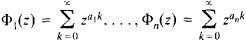each of which converges when |z| < 1 and has a rather simple form, being the sum of the terms of an infinite geometric progression:Consequently,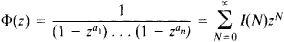where I (N)is the number of solutions of the equation being studied. Euler’s method of generating functions was the source for the Hardy and Littlewood circle method, a far-reaching development of which was I. M. Vinogradov’s method of trigonometric sums.

Another problem of number theory that stimulated the creation of a powerful method was the problem of prime numbers. In proving Euclid’s theorem that the number of prime numbers is infinite, Euler considered the product over all prime numbers p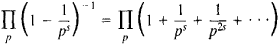for s > 1. This product converges, and if it is expanded, by virtue of the unique factorization of the natural numbers into prime factors. it turns out that this product is equal to the sum of the series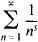whence Euler’s identity follows: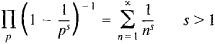When s = 1 the series on the right diverges (a harmonic series), and consequently Euclid’s theorem follows from Euler’s identity. Euler’s idea thus became the basis for the modern theories of the zeta function (seeZETA FUNCTION). Euler and C. Goldbach contributed the first formulations of additive problems, that is, problems connected with addition, involving prime numbers.

By the mid-19th century, the theory of numbers was basically constructed through the efforts of K. Gauss, J. Lagrange, A. Legendre, P. Dirichlet, P. L. Chebyshev, J. Liouville, and E. Kummer.

Gauss created the theory of congruences, also known as the arithmetic of residue classes, which was used to prove the theorem that a prime number is the sum of two squares if and only if it has the form 4n + 1, and the theorem of the representability of every natural number as the sum of four squares of integers. Furthermore, the theory of congruences led to the important number-theoretic concepts of character and trigonometric sum (seeCHARACTER). The Legendre symbol is the simplest character (seeLEGENDRE SYMBOL).

Gauss studied the properties of quadratic residues and nonresidues (seeQUADRATIC RESIDUE). The fundamental theorem in this area is the quadratic reciprocity law, in proving which Gauss considered finite sums of the typewhere a is an integer. Sums of this n = 1 type and their generalizations have come to be called trigonometric sums, since by virtue of Euler’s formula eiϕ = cos ϕ + i sin ϕ they can be represented as the sum of sines and cosines.

Gauss, and later Dirichlet, continuing Euler’s studies, created the theory of quadratic forms, in other words, the theory of the representation of natural numbers by forms of the type ax2 + 2bxy + cy2, where a, b, and c are integers.

Gauss and Dirichlet were the first to consider the problem of the number of lattice points in regions on a plane. Gauss proved that the number of lattice points in the circle X2 + Y2R2 is equal to πR2 + O(R), and Dirichlet in turn proved that the number of lattice points with positive coordinates under the hyperbola xy = N is equal to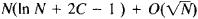, where C is Euler’s constant. Generalizations of these two propositions and the finding of optimal remainders in the formulas written above (Gauss’ problem of lattice points in a circle and Dirichlet’s problem of divisors) were the source for a major chapter in number theory.

Theorems on the infinite number of prime numbers in arithmetic progressions of the type 4k ± 1 and 6k ± 1 had long been known, but Dirichlet was the first to prove the general theorem that there is an infinite number of primes in progressions of the type

nk + l n = 0, 1, 2, …

where k (the difference of the progression) and I (its first term) are relatively prime. Dirichlet considered the following analogue of Euler’s product over all primes: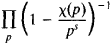Here X(p) is not equal to zero and is periodic X(n + k) = X(n) with a period k and completely multiplicative, that is, X(nm) = X(n)X(m) for any integral n and m. This function is called a Dirichlet character. Dirichlet characters can be used to “cut out” an arithmetic progression. For every natural k there are ϕ(k) Dirichlet characters [ϕ(k) is Euler’s ϕ-function]. If the sum of the numbers X(n) over all possible characters corresponding to k is considered, it will be equal to ϕ(k) if n upon division by k gives a remainder of 1; otherwise it is equal to 0. If s > 1, an analogue of Euler’s identity is obtained:where the series on the right is a Dirichlet series (seeDIRICHLET SERIES). By studying the behavior of such series as s → 1 + 0, Dirichlet proved his theorem of the infinite number of prime numbers in an arithmetic progression.

Dirichlet characters are of considerable importance in both number theory itself and other branches of mathematics, such as algebra and topology, while Dirichlet series constitute a major branch of the modern theory of functions.

A new approach to the problem of the distribution of prime numbers was proposed by Chebyshev. Let π(X)be the number of prime numbers that do not exceed X. Euclid’s theorem asserts that π(X)→ + ∞ as X → + ∞. Chebyshev proved the more exact result thatwhere a > ½ In 2 and b < 2 In 2, and that if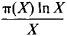tends to a limit as X → ∞, then this limit is equal to 1. Chebyshev also made another discovery in the theory of prime numbers. It had been noted in calculations that a prime number lies in the interval (X, 2X), X ≥ 2,a hypothesis known as Bertrand’s postulate. Chebyshev proved Bertrand’s hypothesis in 1852 but obtained a more exact result by decreasing the length of the interval considered. Thus, together with the problem of twin primes, that is, the problem of the least difference pn + 1Pn the problem of finding upper bounds for this difference arose and began to be solved.

The study of indeterminate equations and, first and foremost, of Fermat’s equation led to the creation of a new branch of number theory—the theory of algebraic numbers. Attempting to prove Fermat’s last theorem, Kummer arrived at the equation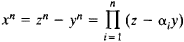where the αi are the n th roots of unity. Considering numbers of the type z + αiy, where z and y are integers, as “new integers,” Kummer constructed the arithmetic of integers of the algebraic number field generated by αi that is, the set of numbers that is obtained from αi by applying all four arithmetical operations to it. If the theorem of the unique factorization of integers into prime factors were valid in such a field, then the above equation would yield a contradiction. However, this is not always the case. In order to preserve the validity of the theorem, Kummer introduced ideal factors. A number of problems arose the solution of which led to the theory of algebraic numbers, with its many new concepts and results.

A new branch in number theory—the study of the arithmetic of the number line—emerged with the study of the properties of integers. Euler had already noted that the square roots of integers and the logarithms of integers fundamentally differ from one another. This fact was given an exact mathematical formulation after the work of Liouville (1844), who introduced the concepts of algebraic number and transcendental number (seeALGEBRAIC NUMBER and TRANSCENDENTAL NUMBER). It turns out that algebraic numbers are approximated “poorly” by rational fractions. Liouville proved that if an algebraic number is a root of an equation of degree n, then it is impossible to approximate it substantially closer than to within Qn by fractions of the type P/Q, where P and Q are relatively prime integers (Liouville’s theorem). From here directly follows the existence of an infinite number of non-algebraic numbers, which came to be called transcendental numbers. For example, the number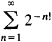will be such a number. However, the question of whether specific numbers are algebraic or transcendental is difficult, and the classical constants π and e were the first to be so examined. Number theory continued to develop in many directions in the late 19th and early 20th centuries. General methods applicable to a broad range of problems, sometimes quite far removed from the original problems, were devised to solve individual problems. The methods and concepts developed often stimulated the development of new fields.

Two trends emerged in the theory of algebraic numbers: the study of specific numbers with a view to proving their transcendentality, and the study of the degree of approximation of algebraic numbers by rational or algebraic numbers. In the first trend, general methods were devised by C. Hermite (1873), who proved the transcendentality of the number e, and by the German mathematician C. L. F. von Lindemann, who proved the transcendentality of the number u and thus solved the problem of squaring the circle. In the second trend, A. Thue proposed (1909) a method of proving that in Liouville’s inequality an algebraic number cannot be approached substantially closer than to within Qn/2. A consequence of this was Thue’s theorem on the finiteness of the number of solutions in integers x and y of the equation

a0xn + a1xn–1 + . . . + an–1xyn–1 + anyn = A

where a0, a1, . . ., an and A are integers and n≥ 3.

The further study of prime numbers led to a new method in number theory connected with the function ζ(s). B. Riemann proved that the zeta function ζ(s)can be continued analytically to the entire complex plane, is analytic at every point of the plane except s = 1, where it has a pole of order 1 with a residue of 1, and satisfies the functional equation ξ(s) = ξ(1 – s)with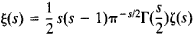and Γ(s)the gamma function. The zeta function has infinitely many zeros in the strip 0 ≤ Re s ≤ 1 (these zeros are said to be nontrivial, and the strip is called the critical strip). Riemann established the close relationship between the nontrivial zeros of ζ(s)and the asymptotic behavior of π(x). The study of the asymptotic formula for Chebyshev’s functionwhere Λ(n) = In p if n = pk and Λ(n) = 0 if npk, is equivalent to the same problem for the function π(x). The function Ψ(x) can be expressed in terms of the integral of the generating function ζ’(s)/ζ(s)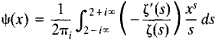Riemann advanced the hypothesis that all nontrivial zeros of ζ(s) lie on the line Re s = ½, from which it follows that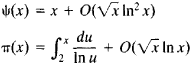The Riemann hypothesis follows from the validity of any one of the last two equations. Dirichlet’s L series were studied in a similar manner. In 1896, C. de La Vallée Poussin and J. Hadamard proved that ζ(s) ≠ 0 in the region Re s ≥ 1, whence followed the formula (the asymptotic law of the distribution of prime numbers)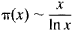Furthermore, La Vallée Poussin proved that ζ(s) ≠ 0 in the regionand that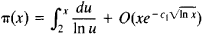where c and c1 are positive constants. He also obtained the same result for prime numbers in arithmetic progressions: if π(x, k, I)is the number of primes of the type kn + l, nx, and k and I are relatively prime, then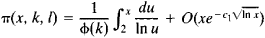The method of obtaining asymptotic formulas for π(x) Ψ(x) and π(x, k, I), which is called the complex integration method, has found numerous applications. The basis of this method is the formula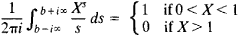The theory of quadratic forms, originated by Euler, Gauss, and Dirichlet, was further developed by A. N. Korkin, E. I. Zolotarev, and A. A. Markov. In particular, Korkin and Zolotarev proved the theorem that the variables of any positive quaternary quadratic form with determinant D can be assigned integral values such that the value of the form will not exceed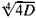, and there exist forms whose minima are equal to. The following is an example of such a form: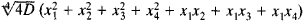Markov’s research dealt with the minima of binary quadratic forms with positive determinant and led to a number of new discoveries.

The problems of lattice points in regions on a plane were developed further in the work of G. F. Voronoi, who devised (1903) a method to prove that the remainder term in Dirichlet’s asymptotic formula for the number of lattice points under a hyperbola is of the order of the cube root of the main term. Later (1966), W. Sierpinski applied Voronoi’s method to Gauss’ problem of lattice points in a circle, with the same result. At the same time, attempts were undertaken to find solutions of the additive problems of number theory, particularly the Waring problem, solved by D. Hubert in 1909 (seeWARING PROBLEM).

The second, third, and fourth decades of the 20th century were exceptionally rich in new ideas and methods in number theory. H. Weyl, in solving problems connected with the stability of the solar system, arrived at the concept of the uniform distribution of the fractional parts of functions on the integers: the fractional parts of the real-valued function F(x)are said to be distributed uniformly over [0, 1) for x = 1, 2, 3, . . . if the number of times the fractional parts of F(x) occur in any interval of [0, 1) is proportional to the length of the interval. Weyl proved that for a uniform distribution of the fractional parts of F(x), it is necessary and sufficient that the following equation be fulfilled for any fixed |m| > 0:He obtained nontrivial estimates of |S(F)x| in the case where F(x) is a polynomial whose leading coefficient is an irrational number. Vinogradov, studying the distribution of the values of the Legendre symbol on segments of short length compared with the modulus, proved (1914) the inequality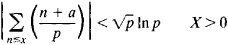from which it follows that the numbers of quadratic residues and nonresidues on any segment whose length is slightly greater thanIn p are asymptotically equal. Furthermore, he advanced the hypothesis that this would be true when X > pε, where ε > 0 is an arbitrarily small number. In 1917, Vinogradov proved that the number of lattice points in the region 0 < yf(x), a < xb, under certain restrictions on the order of growth of the second derivative of f(x), is equal to the area of this region to within a term of the order of the cube root of the main term. The Czech mathematician V. Jarník later established that under the assumptions made with respect to f(x), the accuracy of this formula cannot be improved significantly.

The Norwegian mathematician V. Brun proved (1919) a number of theorems that in a sense approached the problem of twin primes and Euler’s problem. Specifically, he proved the infinite-ness of the number of pairs u1 and u2 such that u2u1 = 2 and such that the number of prime divisors u1 and u2 does not exceed nine; he also proved the solvability of the equation u1 + u2 = 2N, under the same conditions on u1 and u2

G. Hardy and J. Littlewood published (1922–23) a series of monographs under the general title Partitio numerorum, in which they developed a general method of solving additive problems of number theory, which subsequently came to be called the circle method. This method, using the Waring problem as an example, consists in the following. Let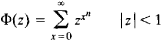then,Here Ik (N) is the number of solutions of Waring’s equation and is given byHardy and Littlewood studied the last integral as R→ 1 – 0. The circle of integration is broken in a specific way into “major” and “minor” arcs (hence the method’s name). Here, the integrals over “major” arcs give the main term of the asymptotic formula for Ik (N), and the integrals over the “minor” arcs give the remainder term. The result is the asymptotic formula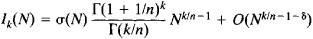where σ(N) is a certain “singular series,” σ(N) ≥ c > 0, δ > 0, and k ≥(n – 2)2n –1 + 5. Using this method, Hardy and Little-wood obtained several results. They obtained a new solution of the Waring problem, a solution in a form more exact than Hubert’s solution. They also obtained a conditional solution of the Goldbach problem and formulated and wrote out hypothetical formulas for the number of solutions of a large number of equations with prime numbers.

In the early 1930’s, Vinogradov developed the method of trigonometric sums, which made it possible to solve many problems in number theory. For example, working on the Waring problem, Vinogradov observed (1929) that the Hardy and Littlewood result would be much simpler if trigonometric sums of the type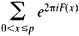where F(x)is a real function, were considered instead of generating functions and if the following relation were used: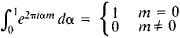where m is an integer. Then, Ik (N) in the Waring problem will be written aswhereThen, the integration interval [0, 1] is divided by rational irreducible fractions of the type a/b, 0 ≤ a < b ≤ т where т is a parameter that depends on N, into subintervals similar to the “major” and “minor” arcs of the circle method. Intervals corresponding to fractions with small denominators and the sum of the integrals over them give the main term of the asymptotic formula for Ik (N). Other intervals correspond to minor arcs; for them, Vinogradov estimated |S(α)| by Weyl’s method and obtained the remainder term. Other problems of number theory also reduce to trigonometric sums, such as the problem of the distribution of fractional parts of functions, the problem of lattice points in regions on a plane and in space, and the problem of the order of growth of the zeta function in the critical strip. What is important in such problems is whether a more exact evaluation of the modulus of a trigonometric sum is possible.

Vinogradov proposed two methods of estimating trigonometric sums. The first method (1934) made it possible to obtain new estimates of Weyl sums, and the current estimates were obtained by way of this method. In addition, an asymptotic formula was derived in the Waring problem for k ≥ 4n2 In n, and it was proved that no more than 3n In n + 11 terms suffice for Waring’s equation to be solvable when N ≥ N0 (n). A new remainder term was also obtained in the asymptotic formulas for π(x) and Ψ(x)(Vinogradov, 1957) of order

O(xec(In x)0.6) c > 0

and the Hilbert-Kamke problem was solved (K. K. Mardzhanishvili, 1953).

Vinogradov’s second method (1937) made it possible to evaluate trigonometric sums in which summation is carried out over prime numbers: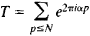This led to the proof of the asymptotic formula for the number of representations of an odd number by the sum of three primes, from which it followed that all sufficiently large odd numbers are the sum of three primes. The Goldbach problem was thus solved (seeGOLDBACH PROBLEM). Vinogradov’s second method also led to the solution of other general problems of number theory, such as the Waring problem in prime numbers and the problem of the distribution of quadratic residues and nonresidues in sequences of the type p + a, where p assumes prime values.

The development of Thue’s ideas (construction of an auxiliary polynomial with a root of high multiplicity) and G. Pólya’s ideas (United States; an entire analytic function that assumes integral values at positive lattice points and that grows more slowly than 2γ|S|, γ <1 is a polynomial) led A. O. Gel’fond and the German mathematician T. Schneider (1934) to the solution of Hubert’s seventh problem, which asserts the transcendentality of numbers of the type αβ, where α ≠ 0, 1 and β is an algebraic number of degree ≥2. K. Siegel proved a series of theorems on the transcendentality of the values of functions of the type ex (E functions) at algebraic points.

In algebraic number theory, a number of theorems that generalize theorems of the theory of integers to the integers of algebraic number fields have been proved; some of them have also led to purely arithmetic results, including the theory of the representation of numbers by complete and incomplete decomposable forms (Pell’s equation is the simplest of these problems). The theory of solutions of congruences of two or more variables has also been developed. It follows, for example, from this theory that the congruence

F(x, y) ≡ 0 (mod p

where F is an absolutely irreducible polynomial, hassolutions (the Hasse-Weyl theorem).

Between the late 1940’s and the present (1978), many papers have appeared in number theory in quite varied areas. Research is being conducted in classical number theory and in various new branches of number theory. The Soviet mathematicians B. N. Delone and D. K. Faddeev investigated in detail (1940) the Diophantine equation x3ay3 = 1. In the theory of the Riemann zeta function, A. Selberg (Norway, 1942) proved that a nonzero fraction of all zeros of ζ(s) lies on the critical line Re s = ½. Iu. V. Linnik proved that the smallest prime number in an arithmetic progression with a difference k does not exceed kc, where c is a constant, and worked out the dispersion method (1958–61), which he used to derive the asymptotic formula for the number of representations of a natural number N by the sum of a prime number and two squares (the Hardy-Littlewood problem). Linnik also used this method to obtain an asymptotic formula for the number of solutions of an indeterminate equation of the type pa = xy, where pN, xyN, and a is a fixed integer (Titchmarsh’s problem of prime divisors). Vinogradov’s method of trigonometric sums was developed further by Vinogradov himself and by his students. Unsuccessful attempts to prove Riemann’s hypothesis led to a number of methods that obviate it and at the same time make it possible to solve certain problems of number theory that are derived from the hypothesis. These include the problem of estimating the difference pn + lpn = Δn, which reduces to estimating the number of zeros of the zeta function in rectangles of the type σ ≤ Re s ≤ 1, σ > 1/2, and |Im s| ≤ T. It follows from such “density” theorems and the bound on the zeros of ζ(s) obtained on the basis of Vinogradov’s method that pn + 1pn = O(pn0.6). Similar results have also been obtained in the theory of the distribution of prime numbers in arithmetic progressions and in its application to additive problems involving prime numbers.

In the theory of transcendental numbers, the British mathematician K. Roth strengthened (1955) Thue’s method and proved that an algebraic number cannot be approximated by a rational fraction P/Q much more accurately than to within Q–2–ε, where ε > 0 is arbitrarily small; the British mathematician A. Baker obtained (1966) an estimate from below for a linear form of the logarithms of algebraic numbers. This led to an effective proof of Thue’s theorem of the finiteness of the number of solutions of the equation

a0x + a1xn–1y + . . . + an–1xyn–1 + anyn = A

(bounds on these solutions are given) and to an effective strengthening of Liouville’s theorem of the approximation of algebraic numbers by rational fractions. A large number of problems of number theory have yet to be solved, including the problems of twin primes, the infiniteness of prime numbers of the type n1 + 1, lattice points in a circle and under a hyperbola, the distribution of the zeros of the zeta function, and the transcendentality of the numbers π + e and Euler’s constant.

REFERENCES

Vinogradov, I. M. Osnovy teorii chisel, 8th ed. Moscow, 1972.
Vinogradov, I. M. Metod trigonometricheskikh summ v teorii chisel. Moscow, 1971.
Vinogradov, I. M. Osobye varianty metoda trigonometricheskikh summ. Moscow, 1976.
Karatsuba, A. A. Osnovy analiticheskoi teorii chisel. Moscow, 1975.
Borevich, Z. I., and I. R. Shafarevich. Teoriia chisel, 2nd ed. Moscow, 1972.
Davenport, H. Mul’tiplikativnaia teoriia chisel. Moscow, 1971. (Translated from English.)
Chandrasekharan, K. Vvedenie v analiticheskuiu teoriiu chisel. Moscow, 1974. (Translated from English.)
Hasse, H. Lektsii po teorii chisel. Moscow, 1953. (Translated from German.)
Dirichlet, P. G. L. Lektsii po teorii chisel. Moscow-Leningrad, 1936. (Translated from German.)
Titchmarsh, E. C. Teoriia dzeta-funktsii Rimana. Moscow, 1953. (Translated from English.)
Venkov, B. A. Elementarnaia teoriia chisel. Moscow-Leningrad, 1937.

A. A. KARATSUBA

Site: Follow: Share:
Open / Close